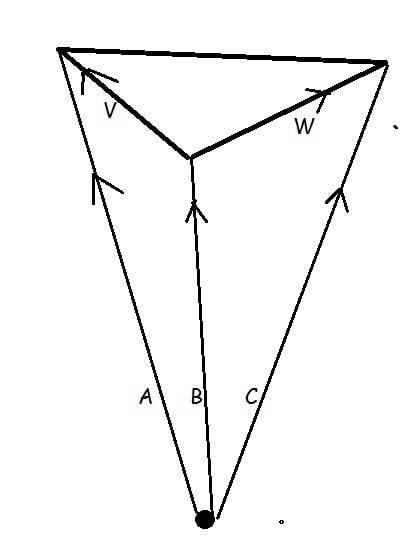# Prove area of triangle is given by cross products of the vertex vectors....

• steele1
steele1

## Homework Statement

The three vectors A, B, and C point from the origin O to the three corners of a triangle. Show that the area of the triangle is given by 1/2|(BxC)+(CxA)+(AxB)|.

## The Attempt at a Solution

I know that the magnitude of the cross product of any two vectors gives the area of the parallelogram formed by those two vectors, and taking half of that would give the area of the triangle. I can see how 1/2|BxC|+1/2|CxA|+1/2|AxB| could give the total area of the triangle formed by the three points, because that would give the sum of the areas of the triangles formed by all 3 possible pairs of vectors which would equal the area of the triangle formed by all 3 vectors. I don't believe that this is the same thing as 1/2|(BxC)+(CxA)+(AxB)|. Not sure where to go from here.

Staff Emeritus

## Homework Statement

The three vectors A, B, and C point from the origin O to the three corners of a triangle. Show that the area of the triangle is given by 1/2|(BxC)+(CxA)+(AxB)|.

## The Attempt at a Solution

I know that the magnitude of the cross product of any two vectors gives the area of the parallelogram formed by those two vectors, and taking half of that would give the area of the triangle. I can see how 1/2|BxC|+1/2|CxA|+1/2|AxB| could give the total area of the triangle formed by the three points, because that would give the sum of the areas of the triangles formed by all 3 possible pairs of vectors which would equal the area of the triangle formed by all 3 vectors. I don't believe that this is the same thing as 1/2|(BxC)+(CxA)+(AxB)|. Not sure where to go from here.

I think you need to draw a picture. If you have a triangle whose sides are the vectors $V$ and $W$, then the area is $\frac{1}{2} V \times W$. But you aren't given $V$ and $W$. Look at this picture:You are not given $V$ and $W$, you are given $A$, $B$ and $C$. Can you compute $V$ and $W$ from $A, B, C$?

steele1
I think you need to draw a picture. If you have a triangle whose sides are the vectors $V$ and $W$, then the area is $\frac{1}{2} V \times W$. But you aren't given $V$ and $W$. Look at this picture:

View attachment 105540

You are not given $V$ and $W$, you are given $A$, $B$ and $C$. Can you compute $V$ and $W$ from $A, B, C$?
I'm honestly not sure how to go about doing that.

Staff Emeritus
I'm honestly not sure how to go about doing that.

Vector addition! In my diagram, if you start at the origin (the black dot) and follow vector $B$, and then follow vector $V$, then you end up at the same place as if you followed vector $A$. This means:

$B+V = A$

You can use that to get $V$ in terms of $A$ and $B$. You can similarly compute $W$ in terms of $B$ and $C$. Got it?

steele1
Vector addition! In my diagram, if you start at the origin (the black dot) and follow vector $B$, and then follow vector $V$, then you end up at the same place as if you followed vector $A$. This means:

$B+V = A$

You can use that to get $V$ in terms of $A$ and $B$. You can similarly compute $W$ in terms of $B$ and $C$. Got it?
haha yup, wow I feel dumb. thought way too into that. So you have V=A-B and W=C-B.

•stevendaryl
steele1
haha yup, wow I feel dumb. thought way too into that. So you have V=A-B and W=C-B.
so 1/2|VxW| would give the area, VxW=(A-B)x(C-B), could you foil and get AxC-AxB-BxC+BxB, and BxB=0, and -AxB=BxA, and -BxC=CxB. So I end up with 1/2|(AxC)+(BxA)+(CxB)| which is all backwards. @stevendaryl